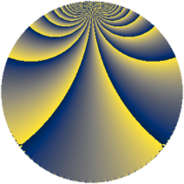# Properties

 Label 684.3.qLevel $684$ Weight $3$ Character orbit 684.q Rep. character $\chi_{684}(163,\cdot)$ Character field $\Q(\zeta_{6})$ Dimension $196$ Sturm bound $360$

# Related objects

## Defining parameters

 Level: $$N$$ $$=$$ $$684 = 2^{2} \cdot 3^{2} \cdot 19$$ Weight: $$k$$ $$=$$ $$3$$ Character orbit: $$[\chi]$$ $$=$$ 684.q (of order $$6$$ and degree $$2$$) Character conductor: $$\operatorname{cond}(\chi)$$ $$=$$ $$76$$ Character field: $$\Q(\zeta_{6})$$ Sturm bound: $$360$$

## Dimensions

The following table gives the dimensions of various subspaces of $$M_{3}(684, [\chi])$$.

Total New Old
Modular forms 496 204 292
Cusp forms 464 196 268
Eisenstein series 32 8 24

## Trace form

 $$196 q + q^{2} + q^{4} + 2 q^{5} + 10 q^{8} + O(q^{10})$$ $$196 q + q^{2} + q^{4} + 2 q^{5} + 10 q^{8} - 12 q^{10} - 10 q^{13} + 12 q^{14} - 15 q^{16} + 26 q^{17} - 48 q^{20} + 33 q^{22} - 452 q^{25} - 16 q^{26} - 42 q^{28} + 2 q^{29} + 21 q^{32} - 48 q^{34} + 72 q^{37} - 108 q^{38} - 10 q^{40} - 66 q^{41} - 15 q^{44} + 292 q^{46} - 1308 q^{49} + 310 q^{50} + 26 q^{52} + 10 q^{53} - 468 q^{56} - 240 q^{58} + 94 q^{61} + 234 q^{62} - 26 q^{64} - 156 q^{65} + 84 q^{68} + 108 q^{70} + 106 q^{73} + 72 q^{74} - 345 q^{76} - 416 q^{77} + 32 q^{80} - 73 q^{82} - 6 q^{85} + 148 q^{86} + 922 q^{88} - 22 q^{89} + 378 q^{92} - 664 q^{94} + 298 q^{97} + 309 q^{98} + O(q^{100})$$

## Decomposition of $$S_{3}^{\mathrm{new}}(684, [\chi])$$ into newform subspaces

The newforms in this space have not yet been added to the LMFDB.

## Decomposition of $$S_{3}^{\mathrm{old}}(684, [\chi])$$ into lower level spaces

$$S_{3}^{\mathrm{old}}(684, [\chi]) \cong$$ $$S_{3}^{\mathrm{new}}(76, [\chi])$$$$^{\oplus 3}$$$$\oplus$$$$S_{3}^{\mathrm{new}}(228, [\chi])$$$$^{\oplus 2}$$